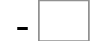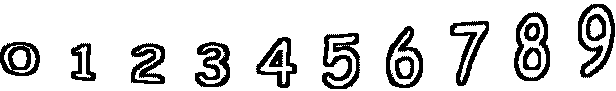45= 7   72= 1583= 21   59= 26   14= 3   16= 5 57= 19   67= 13   71= 33   51= 20